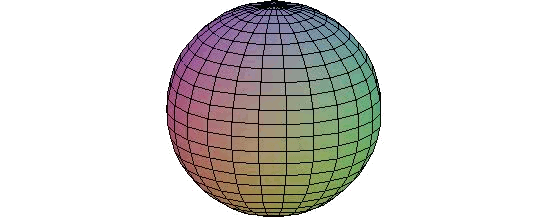# Calculation of surface areas in space

To calculate an area is not much more that integrating function $$1$$ in the given enclosure or surface. Now, we will have a surface in space and, consequently, we will have to restrict the integration of $$\mathbb{R}^3$$ to our surface. This is the so-called surface integral (of the function $$1$$, in our case).

If $$S$$ is a parametric surface, then: $$\text{Area}(S)=\int_S dS=\iint |r_u \times r_v| \ du \ dv$$$where $$r = r (u, v)$$ are the parameters of the surface; $$r_u$$ and $$r_v$$ are the vectors of the partial derivatives with respect to $$u$$ and $$v$$, respectively, and $$|r_u \times r_v|$$ represents the module of the vector product. Therefore, the main difficulty of the calculation is to parametrize the surface. We are going to calculate, to see an example, the surface of a sphere whose radius is $$R$$. We will suppose that it is centred on the origin. Therefore the sphere is given by the equation: $$x^2+y^2+z^2=R^2$$.Taking spherical coordinates, we see that the surface can be written: $$r(\varphi,\theta)=\big( R\sin\varphi\cos\theta,R\sin\varphi\sin\theta, R\cos\varphi\big) \quad \text{ with } \quad \varphi\in[0,\pi] \quad \theta\in[0,2\pi]$$$

Let's calculate now the derivatives of the parametric form and the module of its vector product:

$$\left. \begin{array}{l} r_\varphi=\big(R\cos\theta\cos\varphi, R\sin\theta\cos\varphi,-R\sin\varphi\big) \\ r_\theta=\big(-R\sin\theta\sin\varphi, R\sin\varphi\cos\theta,0\big) \end{array} \right\}$$

$$\begin{array}{lcl} \Rightarrow \ r_\varphi \times r_\theta &=& \begin{vmatrix} i & j & k \\ R\cos\theta\cos\varphi & R\sin\theta\cos\varphi & -R\sin\varphi \\ -R\sin\theta\sin\varphi & R\sin\varphi\cos\theta & 0 \end{vmatrix} \\ &=& \big( R^2\sin^2\varphi\cos\theta, R^2\sin^2\varphi\sin\theta, R^2\sin\varphi\cos\theta\big) \\ \Rightarrow \ |r_\varphi \times r_\theta| &=& \sqrt{R^4\sin^4\varphi\sin^2\theta + R^4\sin^4\varphi\cos^2\varphi + R^4\sin^2\varphi\cos^2\theta} \\ &=& \sqrt{R^4\sin^4\varphi + R^4\sin^2\varphi\cos^2\varphi} = R^2\sin\varphi \end{array}$$

Therefore, we have: $$\begin{array}{rl} \text{Area}(S)=&\int_S dS=\iint |r_\varphi \times r_\theta| \ d\varphi \ d\theta= \int_0^{2\pi}\int_0^{\pi} R^2\sin\varphi \ d\varphi \ d\theta \\ =& R^2 \int_0^{2\pi}[-\cos\varphi]_0^\pi \ d\theta = 2 R^2 2\pi= 4\pi R^2 \end{array}$$\$